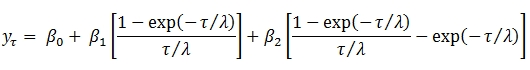# SQL Server Nelson Siegel coefficients for a zero coupon curve

NSCOEF2

Updated: 29 Mar 2013

Use the table-valued function NSCOEF2 to calculate the Nelson Siegel coefficients for a zero coupon curve. Nelson and Siegel suggested calculating the yield curve at a point using this formula:To find the coefficients, the program uses ordinary least squares to calculate the values of B0, B1, and B2 for any value of λ and simply finds the value of λ which has the smallest residual sum of squares in the constraints defined by the input parameters.
Syntax
SELECT * FROM [wctFinancial].[wct].[NSCOEF2](
<@YieldCurve_RangeQuery, nvarchar(max),>
,<@NumSteps, int,>
,<@Tau_min, float,>
,<@Tau_max, float,>
,<@B0_min, float,>
,<@B0_max, float,>
,<@B1_min, float,>
,<@B1_max, float,>
,<@B2_min, float,>
,<@B2_max, float,>)
Arguments
@YieldCurve_RangeQuery
a T-SQL statement, as a string, that specifies the maturities (as measured in years) and their zero coupon rates to be used as in calculating the Nelson Siegel coefficients.
@NumSteps
an integer value that identifies the number of calculations to be done between @Tau_min and @Tau_max. @NumSteps is an expression of type int or of a type that can be implicitly converted to int.
@Tau_min
the lower end of the range of permissible values for tau. @Tau_min is an expression of type float or of a type that can be implicitly converted to float.
@Tau_max
the upper end of the range of permissible values for tau. @Tau_max is an expression of type float or of a type that can be implicitly converted to float.
@B0_min
the lower end of the range of permissible values for B0. @B0_min is an expression of type float or of a type that can be implicitly converted to float.
@B0_max
the upper end of the range of permissible values for B0. @B0_max is an expression of type float or of a type that can be implicitly converted to float.
@B1_min
the lower end of the range of permissible values for B1. @B1_min is an expression of type float or of a type that can be implicitly converted to float.
@B1_max
the upper end of the range of permissible values for B1. @B1_max is an expression of type float or of a type that can be implicitly converted to float.
@B2_min
the lower end of the range of permissible values for B2. @B2_min is an expression of type float or of a type that can be implicitly converted to float.
@B2_max
the upper end of the range of permissible values for B2. @B2_max is an expression of type float or of a type that can be implicitly converted to float.
Return Type
RETURNS TABLE (
[B0] [float] NULL,
[B1] [float] NULL,
[B2] [float] NULL,
[Tau] [float] NULL,
[RMSE] [float] NULL
)

 Column Column Description B0 The first coefficient B1 The second coefficient B2 The third coefficient Tau Tau RMSE The residual sum of squares. SQUARE(SUM(y – y))

Remarks
·         The function is insensitive to order; it does not matter what order the dates and rates are passed in.
·         See NELSONSIEGEL to calculate the interpolated values using the Nelson Siegel coefficients.
·         See NSCOEF for another way to calculate the Nelson Siegel coefficients.
·         If @NumSteps is NULL, @NumSteps = 50.
·         If @Tau_min is NULL, @Tau_min = 0.5
·         If @Tau_max is NULL, @Tau_max = 9.5
·         If @B0_min is NULL, @B0_min = -1
·         If @B0_max is NULL, @B0_max = 1
·         If @B1_min is NULL, @B0_min = -1
·         If @B1_max is NULL, @B1_max = 1
·         If @B2_min is NULL, @B2_min = -1
·         If @B2_max is NULL, @B2_max = 1
Examples
SELECT *
FROM wct.NSCOEF2(
'SELECT 1,0.0028 UNION ALL
SELECT 2,0.0056 UNION ALL
SELECT 3,0.0085 UNION ALL
SELECT 5,0.0164 UNION ALL
SELECT 7,0.0235 UNION ALL
SELECT 10,0.0299 UNION ALL
SELECT 20,0.0382 UNION ALL
SELECT 30,0.0406'
,900
,0.5
,9.5
,-0.5
,0.15
,-0.15
,0.30
,-0.30
,0.30
)
This produces the following result.
B0                  B1                  B2    Tau                   RMSE
------------------ ------------------- ------------------- ------ ----------------------
0.0466551017835633 -0.0408516979746398 -0.0637305177771845 1.6500   9.89520927636627E-07

The following example is illustrative of the process used in the NSCOEF2 function. This example uses the LINEST_q and SeriesFloat functions to do the same calculation.

SELECT TOP(1) tau
,m0
,m1
,m2
,RMSE
FROM (
SELECT k.seriesvalue as tau
,ISNULL(q.stat_name + cast(q.idx as CHAR(1)),'RMSE') as lbl
,q.stat_val
FROM wct.SeriesFloat(0.5,9.5,.01,NULL,NULL) k
CROSS APPLY wct.LINEST_q(
REPLACE('SELECT zr
,(1 - EXP(-cast(mat as float) / @tau)) / (cast(mat as float) / @tau) as x1
,(1 - EXP(-cast(mat as float) / @tau)) / (cast(mat as float) / @tau) - EXP(-cast(mat as float) / @tau) as x2
FROM (
SELECT 1,0.0028 UNION ALL
SELECT 2,0.0056 UNION ALL
SELECT 3,0.0085 UNION ALL
SELECT 5,0.0164 UNION ALL
SELECT 7,0.0235 UNION ALL
SELECT 10,0.0299 UNION ALL
SELECT 20,0.0382 UNION ALL
SELECT 30,0.0406
)n(mat,zr)','@tau',CAST(k.SeriesValue as varchar(max)))
,1
,'True') q
WHERE q.stat_name in('m','ss_resid')) M
PIVOT(
sum(stat_val)
FOR lbl in([m0],[m1],[m2],[RMSE])
) AS PVT
ORDER BY RMSE ASC

This produces the following result.
tau                 m0                  m1                  m2                 RMSE
------ ------------------ ------------------- ------------------- --------------------
1.6500 0.0466551017835633 -0.0408516979746398 -0.0637305177771845 9.89520927636627E-07

### SupportCopyright 2008-2021 Westclintech LLC         Privacy Policy        Terms of Service